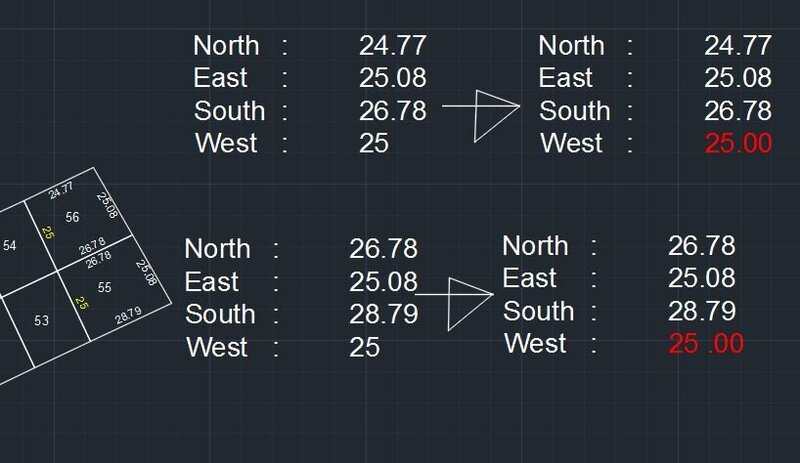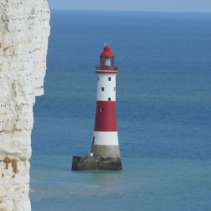# If possible help inserting two numbers after the comma## Recommended PostsHello all
If I have the list  (SETQ LL '( 20 21.3665 22.3265 22 24.5))

If possible TO inserting two numbers after the comma

Let the result look like this  (SETQ LL '( 20.00 21.36 22.32 22.00 24.50))

##### Share on other sitesTry it , I do not understand why you need to add 2 dec. It is as far as I can reach.

```(defun value+2dec (lst )
(setq ll+2-list ())
(foreach l lst
(if (= (type l) 'int)
(progn
(setq l\$(strcat(itoa l)".00"))
(setq ll+2-list (cons ll+2 ll+2-list))
)
(setq ll+2-list (cons  (read(rtos l 2 2)) ll+2-list))
)
)
(reverse ll+2-list)
)

(SETQ LL '( 20 21.3665 22.3265 22 24.5))
(setq ll(value+2dec ll))

```

It return

`(20.0 21.37 22.33 22.0 24.5) `

Hope it help, or give us further explication, about  the WHY  you need it so .

Acad show REALS without unneeded .0 ,  20 is an INTEGER or INT , and 20.0 is a REAL .

•1
##### Share on other sitesUsing rtos can check if any decimals and pad with extra 0's

(setq x 20)
20
: (rtos x 2 2)
: (setq x 20.0)
20.0
: (rtos x 2 2)

: (setq x 20.123)

(rtos x 2 2)
"20.12"

(rtos 20.1 2 2)

##### Share on other sitesIt seem to be  Original Poster WANT, or NEED, or whatever, SEE , all number with 2 decimal , despite it is an INT or a REAL.

Who could know???.

##### Share on other sitesPretty simple algorithm any thing after "." or does it exist.

No "." then strcat ".00"

##### Share on other sitesHI devitg  BIGAL

The reason is that I need to make all values two numbers after the comma
like the photo

```;;;https://www.cadtutor.net/forum/topic/70120-idea-to-determinating-location-lengths-for-polyline/page/2/#comments

;;dlanorh

(defun gc:clockwise-p ( p1 p2 p3 ) (< (sin (- (angle p1 p3) (angle p1 p2))) -1e-14))

(defun rh:polycw-p (obj / tmp rtn)
(if (= (type obj) 'ENAME) (setq obj (vlax-ename->vla-object obj)))
(setq tmp (car (vlax-invoke obj 'offset -0.05)))
(setq rtn (if (> (vlax-get tmp 'area) (vlax-get obj 'area)) T nil))
(vla-delete tmp)
rtn
);end_defun

(defun rh:midpoint ( pt1 pt2 / pt3 ) (setq pt3 (mapcar '(lambda (x y) (/ (+ x y) 2)) pt1 pt2)))

(defun rh:2azimuth ( ang / a_azi)
(if (>= ang (* pi 2.0)) (setq ang (- ang (* pi 2.0))))
(cond ( (and (>= ang 0.0) (< ang (* pi 0.5))) (setq a_azi (- (* pi 0.5) ang)))
( (and (>= ang (* pi 0.5)) (< ang  pi)) (setq a_azi (- (* pi 2.0) (- ang (* pi 0.5)))))
( (and (>= ang  pi) (< ang (* pi 1.5))) (setq a_azi (- (* pi 1.5) (- ang  pi))))
( (and (>= ang (* pi 1.5)) (<= ang (* pi 2.0))) (setq a_azi (- (* pi 0.5) (- ang (* pi 2.0)))))
);end_cond
a_azi
);_end_defun

(defun rh:em_txt ( pt txt lyr ang d72 d73)
(entmakex (list '(0 . "TEXT") '(100 . "AcDbEntity") '(100 . "AcDbText")
(cons 8 lyr) (cons 50 ang) (cons 7 (getvar 'textstyle)) (cons 1 txt)
(cons 10 pt) (cons 40 (getvar 'textsize)) (cons 72 d72) (cons 11 pt) (cons 73  d73)
);end_list
);end_entmakex
);end_defun

(defun rh:em_mtxt (pt txt lyr)
(entmakex (list '(0 . "MTEXT") '(100 . "AcDbEntity") '(100 . "AcDbMText")
(cons 8 lyr) '(50 . 0.0) (cons 7 (getvar 'textstyle))
(cons 1 txt)(cons 10 pt) (cons 40 (* 2.5 (getvar 'textsize)))
);end_list
);end_entmakex
);end_defun

;;BEGIN MAIN ROUTINE
(defun C:BADP ( / *error* tht spcr sel ent obj ep cw cnt nstr sstr estr wstr b vpt ang dst m_pt a_txt l_txt azi i_ang d_pt i_pt)
(setvar 'luprec 2)
(defun *error* ( msg )
(if (not (wcmatch (strcase msg) "*BREAK*,*CANCEL*,*EXIT*")) (princ (strcat "\nOops an Error : " msg " occurred.")))
(princ)
);_end_*error*_defun

(setq tht (getvar 'textsize)
spcr "\U+0009"
);end_setq

(while (setq sel (entsel "\rSelect Polyline Entity to Label : "))
(setq ent (car sel)
obj (vlax-ename->vla-object ent)
ep (vlax-curve-getendparam ent)
cw (rh:polycw-p obj)
cnt 0
nstr "North\U+0009 : "
sstr "South\U+0009 : "
estr "East\U+0009 : "
wstr "West\U+0009 : "
);end_setq

(while (< cnt ep)
(setq b (vla-getbulge obj cnt)
vpt (vlax-curve-getpointatparam ent cnt)
);end_setq
(cond ( (zerop b)
(setq ang (angle '(0.0 0.0 0.0) (vlax-curve-getfirstderiv ent (+ cnt 0.5)))
dst (- (vlax-curve-getdistatparam ent (1+ cnt)) (vlax-curve-getdistatparam ent cnt))
m_pt (vlax-curve-getpointatparam ent (+ cnt 0.5))
);end_setq
)
(t
(setq ang (angle vpt (vlax-curve-getpointatparam ent (1+ cnt)))
dst (- (vlax-curve-getdistatparam ent (1+ cnt)) (vlax-curve-getdistatparam ent cnt))
m_pt (rh:midpoint vpt (vlax-curve-getpointatparam ent (1+ cnt)))
);end_setq
)
);end_cond

(setq l_txt (strcat (rtos dst (getvar 'lunits) 2))
azi (rh:2azimuth ang)
);_end_setq

(cond ( (and (> azi (* pi 0.5)) (<= azi pi)) (if cw (setq estr (strcat estr spcr " " l_txt " ")) (setq wstr (strcat wstr spcr " " l_txt " "))))
( (and (> azi pi) (<= azi (* pi 1.5))) (if cw (setq sstr (strcat sstr spcr " " l_txt " ")) (setq nstr (strcat nstr spcr " " l_txt " "))))
( (and (> azi (* pi 1.5)) (<= azi (* pi 2.0))) (if cw (setq wstr (strcat wstr spcr " " l_txt " ")) (setq estr (strcat estr spcr " " l_txt " "))))
( (and (> azi 0.0) (<= azi (* pi 0.5))) (if cw (setq nstr (strcat nstr spcr " " l_txt " ")) (setq sstr (strcat sstr spcr " " l_txt " "))))
);end_cond

(setq i_ang ang cnt (1+ cnt))
(if (and (>= ang (* pi 0.5)) (< ang (* pi 1.5))) (setq i_ang (- ang pi)))
(setq d_pt (polar m_pt (- i_ang (* pi 0.5)) (* tht 0.3)))
(cond ( (not cw)
(cond ( (gc:clockwise-p vpt m_pt d_pt)
(setq d_pt (polar m_pt (+ i_ang (* pi 0.5)) (* tht 0.3)))
(rh:em_txt d_pt l_txt (getvar 'clayer) i_ang 1 1)
)
(t (rh:em_txt d_pt l_txt (getvar 'clayer) i_ang 1 3))
)
)
(t
(cond ( (not (gc:clockwise-p vpt m_pt d_pt))
(setq d_pt (polar m_pt (+ i_ang (* pi 0.5)) (* tht 0.3)))
(rh:em_txt d_pt l_txt (getvar 'clayer) i_ang 1 1)
)
(t (rh:em_txt d_pt l_txt (getvar 'clayer) i_ang 1 3))
)
)
);end_cond
);_end_while
(initget 1)
(setq i_pt (getpoint "\nSelect Text Insertion Point : "))
(setq a_txt (strcat nstr "\\P" estr "\\P" sstr "\\P" wstr))
(rh:em_mtxt i_pt a_txt (getvar 'clayer))
);end_while
(princ)
);_end_defun
(princ)```##### Share on other sitesAttached is updated lisp to handle cases up to 2 decimal places.

•1
##### Share on other sitesIt looks like trailing zeroes are being suppressed. Perhaps examination of the system variable DIMZIN would have avoided your troubles in the first place.

##### Share on other siteshI hosneyalaa, if you uploaded  your sample dwg . , and show the list as String , and not numbers , all would be easy to give  help . with no doubt .

•1
##### Share on other sites10 hours ago, dlanorh said:

Attached is updated lisp to handle cases up to 2 decimal places.

Thank you  dlanorh

you are a great person
Thank you

``` (setq l_txt (strcat (rtos dst (getvar 'lunits) 2))
azi (rh:2azimuth ang)
slst (vl-string->list l_txt)
);_end_setq

(cond ( (not (vl-position 46 slst)) (setq l_txt (strcat l_txt ".00")))
( (= (vl-position 46 slst) (1- (strlen l_txt))) (setq l_txt (strcat l_txt "00")))
( (= (vl-position 46 slst) (- (strlen l_txt) 2)) (setq l_txt (strcat l_txt "0")))
);end_cond```

##### Share on other sitesEldon solution maybe easiest

```(setq x 20.1)
20.1
: DIMZIN
New current value for DIMZIN (0 to 15) <8>:0
: (rtos x 2 2)
"20.10"
: (setq x 20)
20
: (rtos x 2 2)
"20.00"```

•1

## Join the conversation

You can post now and register later. If you have an account, sign in now to post with your account.
Note: Your post will require moderator approval before it will be visible.×   Pasted as rich text.   Restore formatting

Only 75 emoji are allowed.